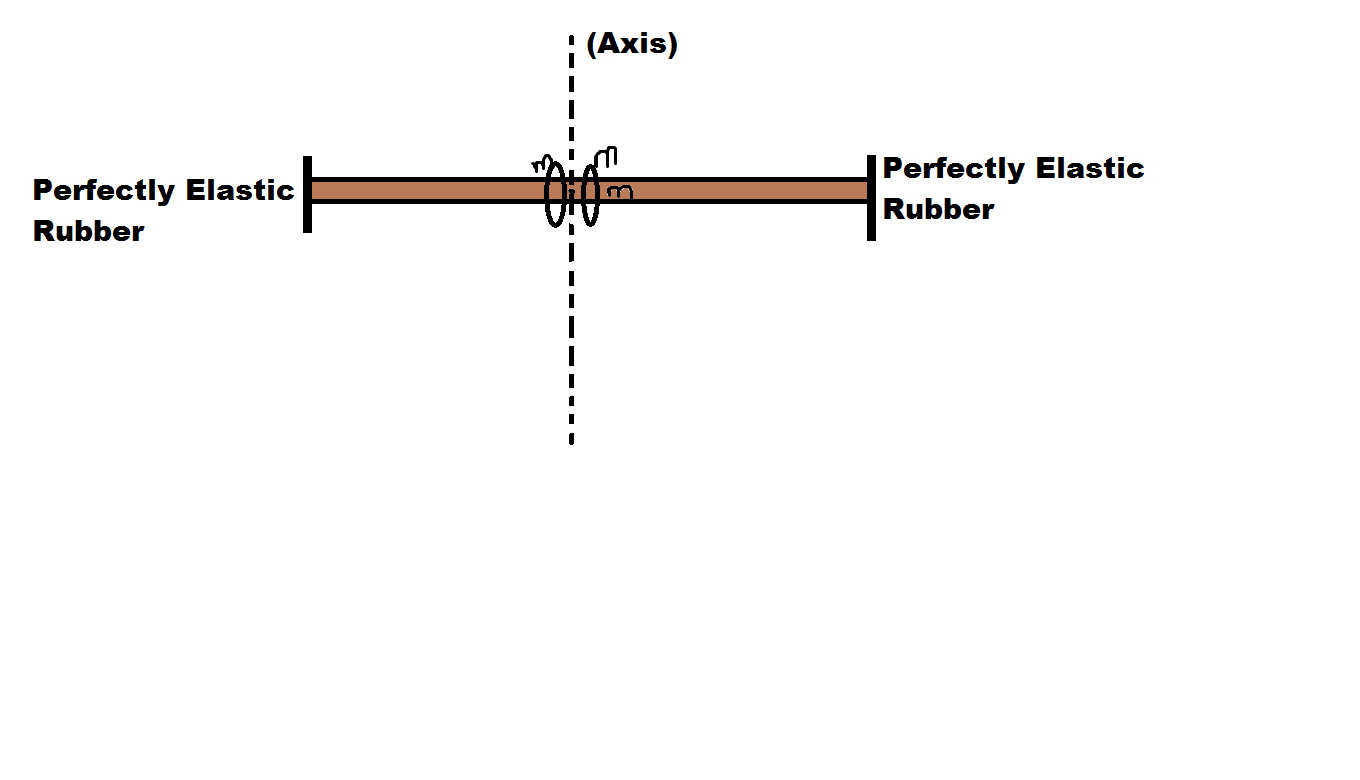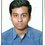# My Elasticity Doubt

Hey guys. I had a question on elasticity which looked something like this.We have small beads of mass say $$m$$ and Mass of rod say $$M$$. Now we need to find the extension in the rod after the beads collide with the the perfectly elastic rubber. Given that it rotates with angular speed $$\omega$$ and

$\text{Young's Modulus} = Y$ and $\text{Area}=A$. [And if you need more variable you can take it. I think the given variables are enough]

No friction is there between the beads.

Now see my solution.

Plan

We have a rotating rod. So we will find out the extension for rotation (Say $x_{1}$) and for the collision say $x_{2}$.

So is it that we need to find out $x_{1}+x_{2}$.

Now we can find $x_{1}$ easily as follows.

Lets take a differential portion of the rod as $dx$.

Now the mass of the differential mass = $dm = \lambda dx$

As rod is uniform we have $\lambda = \dfrac{M}{L}$

$\implies dm= \dfrac{M}{L} dx$.

Now We Stress= $\dfrac{Force}{Area}$

$\implies Stress = \dfrac{dm \omega^2 x}{A}$

$\implies Stress = \dfrac{M \omega^2 dx}{AL}$

$\implies \dfrac{Stress}{Strain} = Y$

$\implies \dfrac{M \omega^2 x^2 dx}{YAL} = d(\Delta x)$

Integrating from $-L \rightarrow +L$ we get

$\implies \Delta x = \dfrac{2M \omega^2 L^2}{3AY} = x_{1}$

Now we have the $x_{2}$ part.

For $x_{2}$. Can we say that the force exerted by each mass on the rod is $m \omega^2 L$ and then

$F= 2 m \omega^2 l$

$\implies \Delta x_{2} = \dfrac{2m \omega^2 L^2}{YA}$

So We can take $x_{1}+x_{2} = \dfrac{2 M \omega^2 L^2 + 6m \omega^2 L^2}{3YA}$

But i am confused if i can take the sum or if the beads play any role in extension or not.

PLEASE HELP!Note by Md Zuhair
3 years, 5 months ago

This discussion board is a place to discuss our Daily Challenges and the math and science related to those challenges. Explanations are more than just a solution — they should explain the steps and thinking strategies that you used to obtain the solution. Comments should further the discussion of math and science.

When posting on Brilliant:

• Use the emojis to react to an explanation, whether you're congratulating a job well done , or just really confused .
• Ask specific questions about the challenge or the steps in somebody's explanation. Well-posed questions can add a lot to the discussion, but posting "I don't understand!" doesn't help anyone.
• Try to contribute something new to the discussion, whether it is an extension, generalization or other idea related to the challenge.
• Stay on topic — we're all here to learn more about math and science, not to hear about your favorite get-rich-quick scheme or current world events.

MarkdownAppears as
*italics* or _italics_ italics
**bold** or __bold__ bold
- bulleted- list
• bulleted
• list
1. numbered2. list
1. numbered
2. list
Note: you must add a full line of space before and after lists for them to show up correctly
paragraph 1paragraph 2

paragraph 1

paragraph 2

[example link](https://brilliant.org)example link
> This is a quote
This is a quote
    # I indented these lines
# 4 spaces, and now they show
# up as a code block.

print "hello world"
# I indented these lines
# 4 spaces, and now they show
# up as a code block.

print "hello world"
MathAppears as
Remember to wrap math in $$ ... $$ or $ ... $ to ensure proper formatting.
2 \times 3 $2 \times 3$
2^{34} $2^{34}$
a_{i-1} $a_{i-1}$
\frac{2}{3} $\frac{2}{3}$
\sqrt{2} $\sqrt{2}$
\sum_{i=1}^3 $\sum_{i=1}^3$
\sin \theta $\sin \theta$
\boxed{123} $\boxed{123}$

## Comments

Sort by:

Top Newest
- 3 years, 5 months ago

Log in to reply

Zuhair.... If we take lengh of the rod to be L then why should we integrate from -L to +L(instead -L/2 to +L/2). I also think the tension in the differential portion also depend on the mass and acceleration of the all small part of the rod to the side of nearer end of the rod . So we have to integrate and find out the total tension contributed to the differential mass as a function of 'distance from axis' and substitute it in Young's equation. I do not think the bead will involve.

- 3 years, 5 months ago

Log in to reply

Oh yes sir. Thats typo error because I was typing without any written peice. So take lenght as 2L. Or else I will just edit it sometime. Can you please provide a solution if you didnt did it this way and your issues abput thte tension. Thanks

- 3 years, 5 months ago

Log in to reply

Otherwise, is the concept right?

- 3 years, 4 months ago

Log in to reply

×

Problem Loading...

Note Loading...

Set Loading...